xIsomorphismEncyclopedia
In abstract algebra
Abstract algebra
Abstract algebra is the subject area of mathematics that studies algebraic structures, such as groups, rings, fields, modules, vector spaces, and algebras...

, an isomorphism (Greek
Greek language
Greek is an independent branch of the Indo-European family of languages. Native to the southern Balkans, it has the longest documented history of any Indo-European language, spanning 34 centuries of written records. Its writing system has been the Greek alphabet for the majority of its history;...

: ἴσος isos "equal", and μορφή morphe "shape") is a mapping
Map (mathematics)
In most of mathematics and in some related technical fields, the term mapping, usually shortened to map, is either a synonym for function, or denotes a particular kind of function which is important in that branch, or denotes something conceptually similar to a function.In graph theory, a map is a...

between objects that shows a relationship between two properties or operations.  If there exists an isomorphism between two structures, the two structures are said to be isomorphic.  In a certain sense, isomorphic structures are structurally identical, if more minute definitional differences are ignored.  More formally, an isomorphism is a bijective map f such that both f and its inverse
Inverse function
In mathematics, an inverse function is a function that undoes another function: If an input x into the function ƒ produces an output y, then putting y into the inverse function g produces the output x, and vice versa. i.e., ƒ=y, and g=x...

f −1 are structure-preserving maps
Map (mathematics)
In most of mathematics and in some related technical fields, the term mapping, usually shortened to map, is either a synonym for function, or denotes a particular kind of function which is important in that branch, or denotes something conceptually similar to a function.In graph theory, a map is a...

between two algebraic structure
Algebraic structure
In abstract algebra, an algebraic structure consists of one or more sets, called underlying sets or carriers or sorts, closed under one or more operations, satisfying some axioms. Abstract algebra is primarily the study of algebraic structures and their properties...

s, that is, they are both homomorphism
Homomorphism
In abstract algebra, a homomorphism is a structure-preserving map between two algebraic structures . The word homomorphism comes from the Greek language: ὁμός meaning "same" and μορφή meaning "shape".- Definition :The definition of homomorphism depends on the type of algebraic structure under...

s.

In category theory
Category theory
Category theory is an area of study in mathematics that examines in an abstract way the properties of particular mathematical concepts, by formalising them as collections of objects and arrows , where these collections satisfy certain basic conditions...

, an isomorphism is a morphism
Morphism
In mathematics, a morphism is an abstraction derived from structure-preserving mappings between two mathematical structures. The notion of morphism recurs in much of contemporary mathematics...

in a category for which there exists an "inverse" with the property that both and

## Purpose

Isomorphisms are studied in mathematics in order to extend insights from one phenomenon to others: if two objects are isomorphic, then any property that is preserved by an isomorphism and that is true of one of the objects, is also true of the other. If an isomorphism can be found from a relatively unknown part of mathematics into some well studied division of mathematics, where many theorems are already proved, and many methods are already available to find answers, then the function can be used to map whole problems out of unfamiliar territory over to "solid ground" where the problem is easier to understand and work with.

## Practical examples

The following are examples of isomorphisms from ordinary algebra
Algebra
Algebra is the branch of mathematics concerning the study of the rules of operations and relations, and the constructions and concepts arising from them, including terms, polynomials, equations and algebraic structures...

.

• Consider the logarithm
Logarithm
The logarithm of a number is the exponent by which another fixed value, the base, has to be raised to produce that number. For example, the logarithm of 1000 to base 10 is 3, because 1000 is 10 to the power 3: More generally, if x = by, then y is the logarithm of x to base b, and is written...

function: For any fixed base b, the logarithm function logb maps from the positive real number
Real number
In mathematics, a real number is a value that represents a quantity along a continuum, such as -5 , 4/3 , 8.6 , √2 and π...

s R+ onto the real numbers R; formally: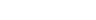This mapping is one-to-one
Injective function
In mathematics, an injective function is a function that preserves distinctness: it never maps distinct elements of its domain to the same element of its codomain. In other words, every element of the function's codomain is mapped to by at most one element of its domain...

and onto
Surjective function
In mathematics, a function f from a set X to a set Y is surjective , or a surjection, if every element y in Y has a corresponding element x in X so that f = y...

, that is, it is a bijection
Bijection
A bijection is a function giving an exact pairing of the elements of two sets. A bijection from the set X to the set Y has an inverse function from Y to X. If X and Y are finite sets, then the existence of a bijection means they have the same number of elements...

from the domain
Domain (mathematics)
In mathematics, the domain of definition or simply the domain of a function is the set of "input" or argument values for which the function is defined...

to the codomain
Codomain
In mathematics, the codomain or target set of a function is the set into which all of the output of the function is constrained to fall. It is the set in the notation...

of the logarithm function.

In addition to being an isomorphism of sets, the logarithm function also preserves certain operations. Specifically, consider the group
Group (mathematics)
In mathematics, a group is an algebraic structure consisting of a set together with an operation that combines any two of its elements to form a third element. To qualify as a group, the set and the operation must satisfy a few conditions called group axioms, namely closure, associativity, identity...

(R+,×) of positive real numbers under ordinary multiplication. The logarithm function obeys the following identity: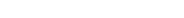But the real numbers under addition also form a group. So the logarithm function is in fact a group isomorphism from the group (R+,×) to the group (R,+).

Logarithms can therefore be used to simplify multiplication of real numbers. By working with logarithms, multiplication of positive real numbers is replaced by addition of logs. This way it is possible to multiply real numbers using a ruler
Ruler
A ruler, sometimes called a rule or line gauge, is an instrument used in geometry, technical drawing, printing and engineering/building to measure distances and/or to rule straight lines...

and a table of logarithms, or using a slide rule
Slide rule
The slide rule, also known colloquially as a slipstick, is a mechanical analog computer. The slide rule is used primarily for multiplication and division, and also for functions such as roots, logarithms and trigonometry, but is not normally used for addition or subtraction.Slide rules come in a...

with a logarithmic scale.

• Consider the group (Z6, +), the integers from 0 to 5 with addition modulo
Modular arithmetic
In mathematics, modular arithmetic is a system of arithmetic for integers, where numbers "wrap around" after they reach a certain value—the modulus....

6. Also consider the group (Z2 × Z3, +), the ordered pairs where the x coordinates can be 0 or 1, and the y coordinates can be 0, 1, or 2, where addition in the x-coordinate is modulo 2 and addition in the y-coordinate is modulo 3.

These structures are isomorphic under addition, if you identify them using the following scheme:
→ 0 → 1 → 2 → 3 → 4 → 5

or in general (a,b) → (3a + 4b) mod 6.

For example note that (1,1) + (1,0) = (0,1), which translates in the other system as 1 + 3 = 4.

Even though these two groups "look" different in that the sets contain different elements, they are indeed isomorphic: their structures are exactly the same. More generally, the direct product
Direct product of groups
In the mathematical field of group theory, the direct product is an operation that takes two groups and and constructs a new group, usually denoted...

of two cyclic group
Cyclic group
In group theory, a cyclic group is a group that can be generated by a single element, in the sense that the group has an element g such that, when written multiplicatively, every element of the group is a power of g .-Definition:A group G is called cyclic if there exists an element g...

s Zm and Zn is isomorphic to Zmn if and only if m and n are coprime
Coprime
In number theory, a branch of mathematics, two integers a and b are said to be coprime or relatively prime if the only positive integer that evenly divides both of them is 1. This is the same thing as their greatest common divisor being 1...

.

### A relation-preserving isomorphism

If one object consists of a set X with a binary relation
Binary relation
In mathematics, a binary relation on a set A is a collection of ordered pairs of elements of A. In other words, it is a subset of the Cartesian product A2 = . More generally, a binary relation between two sets A and B is a subset of...

R and the other object consists of a set Y with a binary relation S then an isomorphism from X to Y is a bijective function such that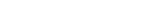S is reflexive
Reflexive relation
In mathematics, a reflexive relation is a binary relation on a set for which every element is related to itself, i.e., a relation ~ on S where x~x holds true for every x in S. For example, ~ could be "is equal to".-Related terms:...

, irreflexive, symmetric
Symmetric relation
In mathematics, a binary relation R over a set X is symmetric if it holds for all a and b in X that if a is related to b then b is related to a.In mathematical notation, this is:...

, antisymmetric
Antisymmetric relation
In mathematics, a binary relation R on a set X is antisymmetric if, for all a and b in Xor, equivalently,In mathematical notation, this is:\forall a, b \in X,\ R \and R \; \Rightarrow \; a = bor, equivalently,...

, asymmetric
Asymmetric relation
Asymmetric often means, simply: not symmetric. In this sense an asymmetric relation is a binary relation which is not a symmetric relation.That is,\lnot....

, transitive
Transitive relation
In mathematics, a binary relation R over a set X is transitive if whenever an element a is related to an element b, and b is in turn related to an element c, then a is also related to c....

, total
Total relation
In mathematics, a binary relation R over a set X is total if for all a and b in X, a is related to b or b is related to a .In mathematical notation, this is\forall a, b \in X,\ a R b \or b R a....

, trichotomous, a partial order, total order
Total order
In set theory, a total order, linear order, simple order, or ordering is a binary relation on some set X. The relation is transitive, antisymmetric, and total...

, strict weak order, total preorder (weak order), an equivalence relation
Equivalence relation
In mathematics, an equivalence relation is a relation that, loosely speaking, partitions a set so that every element of the set is a member of one and only one cell of the partition. Two elements of the set are considered equivalent if and only if they are elements of the same cell...

, or a relation with any other special properties, if and only if R is.

For example, R is an ordering
Order theory
Order theory is a branch of mathematics which investigates our intuitive notion of order using binary relations. It provides a formal framework for describing statements such as "this is less than that" or "this precedes that". This article introduces the field and gives some basic definitions...

≤ and S an ordering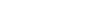, then an isomorphism from X to Y is a bijective function such that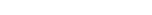Such an isomorphism is called an order isomorphism
Order isomorphism
In the mathematical field of order theory an order isomorphism is a special kind of monotone function that constitutes a suitable notion of isomorphism for partially ordered sets . Whenever two posets are order isomorphic, they can be considered to be "essentially the same" in the sense that one of...

or (less commonly) an isotone isomorphism.

If this is a relation-preserving automorphism
Automorphism
In mathematics, an automorphism is an isomorphism from a mathematical object to itself. It is, in some sense, a symmetry of the object, and a way of mapping the object to itself while preserving all of its structure. The set of all automorphisms of an object forms a group, called the automorphism...

.

### An operation-preserving isomorphism

Suppose that on these sets X and Y, there are two binary operation
Binary operation
In mathematics, a binary operation is a calculation involving two operands, in other words, an operation whose arity is two. Examples include the familiar arithmetic operations of addition, subtraction, multiplication and division....

s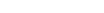and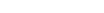that happen to constitute the groups
Group (mathematics)
In mathematics, a group is an algebraic structure consisting of a set together with an operation that combines any two of its elements to form a third element. To qualify as a group, the set and the operation must satisfy a few conditions called group axioms, namely closure, associativity, identity...

(X,) and (Y,). Note that the operators operate on elements from the domain
Domain (mathematics)
In mathematics, the domain of definition or simply the domain of a function is the set of "input" or argument values for which the function is defined...

and range
Range (mathematics)
In mathematics, the range of a function refers to either the codomain or the image of the function, depending upon usage. This ambiguity is illustrated by the function f that maps real numbers to real numbers with f = x^2. Some books say that range of this function is its codomain, the set of all...

, respectively, of the "one-to-one" and "onto" function ƒ. There is an isomorphism from X to Y if the bijective function happens to produce results, that sets up a correspondence between the operatorand the operator.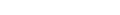for all u, v in X.

## Applications

In abstract algebra
Abstract algebra
Abstract algebra is the subject area of mathematics that studies algebraic structures, such as groups, rings, fields, modules, vector spaces, and algebras...

, two basic isomorphisms are defined:
• Group isomorphism
Group isomorphism
In abstract algebra, a group isomorphism is a function between two groups that sets up a one-to-one correspondence between the elements of the groups in a way that respects the given group operations. If there exists an isomorphism between two groups, then the groups are called isomorphic...

, an isomorphism between groups
Group (mathematics)
In mathematics, a group is an algebraic structure consisting of a set together with an operation that combines any two of its elements to form a third element. To qualify as a group, the set and the operation must satisfy a few conditions called group axioms, namely closure, associativity, identity...

• Ring isomorphism, an isomorphism between rings
Ring (mathematics)
In mathematics, a ring is an algebraic structure consisting of a set together with two binary operations usually called addition and multiplication, where the set is an abelian group under addition and a semigroup under multiplication such that multiplication distributes over addition...

. (Note that isomorphisms between fields
Field (mathematics)
In abstract algebra, a field is a commutative ring whose nonzero elements form a group under multiplication. As such it is an algebraic structure with notions of addition, subtraction, multiplication, and division, satisfying certain axioms...

are actually ring isomorphisms)

Just as the automorphism
Automorphism
In mathematics, an automorphism is an isomorphism from a mathematical object to itself. It is, in some sense, a symmetry of the object, and a way of mapping the object to itself while preserving all of its structure. The set of all automorphisms of an object forms a group, called the automorphism...

s of an algebraic structure
Algebraic structure
In abstract algebra, an algebraic structure consists of one or more sets, called underlying sets or carriers or sorts, closed under one or more operations, satisfying some axioms. Abstract algebra is primarily the study of algebraic structures and their properties...

form a group
Group (mathematics)
In mathematics, a group is an algebraic structure consisting of a set together with an operation that combines any two of its elements to form a third element. To qualify as a group, the set and the operation must satisfy a few conditions called group axioms, namely closure, associativity, identity...

, the isomorphisms between two algebras sharing a common structure form a heap
Heap (mathematics)
In abstract algebra, a heap is a mathematical generalisation of a group. Informally speaking, a heap is obtained from a group by "forgetting" which element is the unit, in the same way that an affine space can be viewed as a vector space in which the 0 element has been "forgotten"...

. Letting a particular isomorphism identify the two structures turns this heap into a group.

In mathematical analysis
Mathematical analysis
Mathematical analysis, which mathematicians refer to simply as analysis, has its beginnings in the rigorous formulation of infinitesimal calculus. It is a branch of pure mathematics that includes the theories of differentiation, integration and measure, limits, infinite series, and analytic functions...

, the Laplace transform is an isomorphism mapping hard differential equations into easier algebra
Algebra
Algebra is the branch of mathematics concerning the study of the rules of operations and relations, and the constructions and concepts arising from them, including terms, polynomials, equations and algebraic structures...

ic equations.

In category theory
Category theory
Category theory is an area of study in mathematics that examines in an abstract way the properties of particular mathematical concepts, by formalising them as collections of objects and arrows , where these collections satisfy certain basic conditions...

, Iet the category
Category (mathematics)
In mathematics, a category is an algebraic structure that comprises "objects" that are linked by "arrows". A category has two basic properties: the ability to compose the arrows associatively and the existence of an identity arrow for each object. A simple example is the category of sets, whose...

C consist of two classes
Class (set theory)
In set theory and its applications throughout mathematics, a class is a collection of sets which can be unambiguously defined by a property that all its members share. The precise definition of "class" depends on foundational context...

, one of objects and the other of morphisms. Then a general definition of isomorphism that covers the previous and many other cases is: an isomorphism is a morphism that has an inverse, i.e. there exists a morphism with and . For example, a bijective linear map is an isomorphism between vector space
Vector space
A vector space is a mathematical structure formed by a collection of vectors: objects that may be added together and multiplied by numbers, called scalars in this context. Scalars are often taken to be real numbers, but one may also consider vector spaces with scalar multiplication by complex...

s, and a bijective continuous function
Continuous function
In mathematics, a continuous function is a function for which, intuitively, "small" changes in the input result in "small" changes in the output. Otherwise, a function is said to be "discontinuous". A continuous function with a continuous inverse function is called "bicontinuous".Continuity of...

whose inverse is also continuous is an isomorphism between topological space
Topological space
Topological spaces are mathematical structures that allow the formal definition of concepts such as convergence, connectedness, and continuity. They appear in virtually every branch of modern mathematics and are a central unifying notion...

s, called a homeomorphism
Homeomorphism
In the mathematical field of topology, a homeomorphism or topological isomorphism or bicontinuous function is a continuous function between topological spaces that has a continuous inverse function. Homeomorphisms are the isomorphisms in the category of topological spaces—that is, they are...

.

In graph theory
Graph theory
In mathematics and computer science, graph theory is the study of graphs, mathematical structures used to model pairwise relations between objects from a certain collection. A "graph" in this context refers to a collection of vertices or 'nodes' and a collection of edges that connect pairs of...

, an isomorphism between two graphs G and H is a bijective map f from the vertices of G to the vertices of H that preserves the "edge structure" in the sense that there is an edge from vertex
Vertex (graph theory)
In graph theory, a vertex or node is the fundamental unit out of which graphs are formed: an undirected graph consists of a set of vertices and a set of edges , while a directed graph consists of a set of vertices and a set of arcs...

u to vertex v in G if and only if there is an edge from ƒ(u) to ƒ(v) in H. See graph isomorphism
Graph isomorphism
In graph theory, an isomorphism of graphs G and H is a bijection between the vertex sets of G and H f \colon V \to V \,\!such that any two vertices u and v of G are adjacent in G if and only if ƒ and ƒ are adjacent in H...

.

In mathematical analysis, an isomorphism between two Hilbert spaces is a bijection preserving addition, scalar multiplication, and inner product.

In early theories of logical atomism
Logical atomism
Logical atomism is a philosophical belief that originated in the early 20th century with the development of analytic philosophy. Its principal exponents were the British philosopher Bertrand Russell, the early work of his Austrian-born pupil and colleague Ludwig Wittgenstein, and his German...

, the formal relationship between facts and true propositions was theorized by Bertrand Russell
Bertrand Russell
Bertrand Arthur William Russell, 3rd Earl Russell, OM, FRS was a British philosopher, logician, mathematician, historian, and social critic. At various points in his life he considered himself a liberal, a socialist, and a pacifist, but he also admitted that he had never been any of these things...

and Ludwig Wittgenstein
Ludwig Wittgenstein
Ludwig Josef Johann Wittgenstein was an Austrian philosopher who worked primarily in logic, the philosophy of mathematics, the philosophy of mind, and the philosophy of language. He was professor in philosophy at the University of Cambridge from 1939 until 1947...

to be isomorphic. An example of this line of thinking can be found in Russell's Introduction to Mathematical Philosophy
Introduction to Mathematical Philosophy
Introduction to Mathematical Philosophy is a book by Bertrand Russell, published in 1919, written in part to exposit in a less technical way the main ideas of his and Whitehead's Principia Mathematica , including the theory of descriptions....

.

In cybernetics
Cybernetics
Cybernetics is the interdisciplinary study of the structure of regulatory systems. Cybernetics is closely related to information theory, control theory and systems theory, at least in its first-order form...

, the Good Regulator
Good Regulator
The Good Regulator is a theorem due to Roger C. Conant and W. Ross Ashby that is central to cybernetics. It is stated "Every Good Regulator of a system must be a model of that system"....

or Conant-Ashby theorem is stated "Every Good Regulator of a system must be a model of that system". Whether regulated or self-regulating an isomorphism is required between regulator part and the processing part of the system.

## Relation with equality

In certain areas of mathematics, notably category theory
Category theory
Category theory is an area of study in mathematics that examines in an abstract way the properties of particular mathematical concepts, by formalising them as collections of objects and arrows , where these collections satisfy certain basic conditions...

, it is valuable to distinguish between equality on the one hand and isomorphism on the other. Equality is when two objects are "literally the same", while isomorphism is when two objects "can be made to correspond via an isomorphism". For example, the sets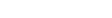and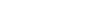are equal – they are two different presentations (one in set builder notation, one by an enumeration) of the same subset of the integers. By contrast, the sets {A,B,C} and {1,2,3} are not equal – the first has elements that are letters, while the second has elements that are numbers. These are isomorphic as sets, since finite sets are determined up to isomorphism by their cardinality (number of elements) and these both have three elements, but there are many choices of isomorphism – one isomorphism is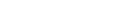while another is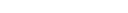and no one isomorphism is better than any other.The careful reader may note that A, B, C have a conventional order, namely alphabetical order, and similarly 1, 2, 3 have the order from the integers, and thus in some sense there is a "natural" isomorphism, namely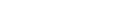This intuition can be formalized by saying that any two finite ordered sets
Ordered set
In order theory in mathematics, a set with a binary relation R on its elements that is reflexive , antisymmetric and transitive is described as a partially ordered set or poset...

of the same cardinality have a natural isomorphism, by sending the least element of the first to the least element of the second, the least element of what remains in the first to the least element of what remains in the second, and so forth, but conversely two finite unordered sets are not naturally isomorphic because there is more than one choice of map – except if the cardinality is 0 or 1, where there is a unique choice.
In fact, there are precisely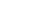different isomorphism between two sets with three elements. This is equal to the number of automorphism
Automorphism
In mathematics, an automorphism is an isomorphism from a mathematical object to itself. It is, in some sense, a symmetry of the object, and a way of mapping the object to itself while preserving all of its structure. The set of all automorphisms of an object forms a group, called the automorphism...

s of a given three element set (which in turn is equal to the order of the symmetric group
Symmetric group
In mathematics, the symmetric group Sn on a finite set of n symbols is the group whose elements are all the permutations of the n symbols, and whose group operation is the composition of such permutations, which are treated as bijective functions from the set of symbols to itself...

on three letters), and more generally one has that the set of isomorphisms between two objects, denoted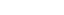is a torsor for the automorphism group of A,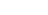and also a torsor for the automorphism group of B. In fact, automorphisms of an object are a key reason to be concerned with the distinction between isomorphism and equality, as demonstrated in the effect of change of basis on the identification of a vector space with its dual or with its double dual, as elaborated in the sequel.
Thus one cannot identify these two sets: one can choose an isomorphism between them, but any statement that identifies these two sets depends on the choice of isomorphism.

A motivating example is the distinction between a finite-dimensional vector space V and its dual space
Dual space
In mathematics, any vector space, V, has a corresponding dual vector space consisting of all linear functionals on V. Dual vector spaces defined on finite-dimensional vector spaces can be used for defining tensors which are studied in tensor algebra...

} of linear maps from V to its field of scalars K.
These spaces have the same dimension, and thus are isomorphic as abstract vector spaces (since algebraically, vector spaces are classified by dimension, just as sets are classified by cardinality), but there is no "natural" choice of isomorphism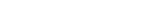.
If one chooses a basis for V, then this yields an isomorphism: For all ,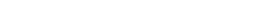.

This corresponds to transforming a column vector (element of V) to a row vector (element of V*) by transpose
Transpose
In linear algebra, the transpose of a matrix A is another matrix AT created by any one of the following equivalent actions:...

, but a different choice of basis gives a different isomorphism: the isomorphism "depends on the choice of basis".
More subtly, there is a map from a vector space V to its double dual } that does not depend on the choice of basis: For all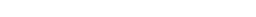.

This leads to a third notion, that of a natural isomorphism: while V and V** are different sets, there is a "natural" choice of isomorphism between them.
This intuitive notion of "an isomorphism that does not depend on an arbitrary choice" is formalized in the notion of a natural transformation
Natural transformation
In category theory, a branch of mathematics, a natural transformation provides a way of transforming one functor into another while respecting the internal structure of the categories involved. Hence, a natural transformation can be considered to be a "morphism of functors". Indeed this intuition...

; briefly, that one may consistently identify, or more generally map from, a vector space to its double dual,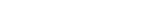, for any vector space in a consistent way.
Formalizing this intuition is a motivation for the development of category theory.

If one wishes to draw a distinction between an arbitrary isomorphism (one that depends on a choice) and a natural isomorphism (one that can be done consistently), one may write for an unnatural isomorphism and ≅ for a natural isomorphism, as in and
This convention is not universally followed, and authors who wish to distinguish between unnatural isomorphisms and natural isomorphisms will generally explicitly state the distinction.

Generally, saying that two objects are equal is reserved for when there is a notion of a larger (ambient) space that these objects live in. Most often, one speaks of equality of two subsets of a given set (as in the integer set example above), but not of two objects abstractly presented. For example, the 2-dimensional unit sphere in 3-dimensional space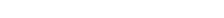and the Riemann sphere
Riemann sphere
In mathematics, the Riemann sphere , named after the 19th century mathematician Bernhard Riemann, is the sphere obtained from the complex plane by adding a point at infinity...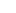which can be presented as the one-point compactification of the complex plane } or as the complex projective line
Projective line
In mathematics, a projective line is a one-dimensional projective space. The projective line over a field K, denoted P1, may be defined as the set of one-dimensional subspaces of the two-dimensional vector space K2 .For the generalisation to the projective line over an associative ring, see...

(a quotient space
Quotient space
In topology and related areas of mathematics, a quotient space is, intuitively speaking, the result of identifying or "gluing together" certain points of a given space. The points to be identified are specified by an equivalence relation...

)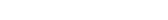are three different descriptions for a mathematical object, all of which are isomorphic, but not equal because they are not all subsets of a single space: the first is a subset of R3, the second is 2Being precise, the identification of the complex numbers with the real plane,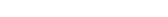depends on a choice of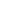one can just as easily choose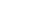, which yields a different identification – formally, complex conjugation is an automorphism – but in practice one often assumes that one has made such an identification.
plus an additional point, and the third is a subquotient
Subquotient
In the mathematical fields of category theory and abstract algebra, a subquotient is a quotient object of a subobject. Subquotients are particularly important in group theory, where they are also known as sections, and in abelian categories....

of C2

• Epimorphism
Epimorphism
In category theory, an epimorphism is a morphism f : X → Y which is right-cancellative in the sense that, for all morphisms ,...

• Heap (mathematics)
Heap (mathematics)
In abstract algebra, a heap is a mathematical generalisation of a group. Informally speaking, a heap is obtained from a group by "forgetting" which element is the unit, in the same way that an affine space can be viewed as a vector space in which the 0 element has been "forgotten"...

• Isometry
Isometry
In mathematics, an isometry is a distance-preserving map between metric spaces. Geometric figures which can be related by an isometry are called congruent.Isometries are often used in constructions where one space is embedded in another space...

• Isomorphism class
Isomorphism class
An isomorphism class is a collection of mathematical objects isomorphic to each other.Isomorphism classes are often defined if the exact identity of the elements of the set is considered irrelevant, and the properties of the structure of the mathematical object are studied. Examples of this are...

• Monomorphism
Monomorphism
In the context of abstract algebra or universal algebra, a monomorphism is an injective homomorphism. A monomorphism from X to Y is often denoted with the notation X \hookrightarrow Y....

• Morphism
Morphism
In mathematics, a morphism is an abstraction derived from structure-preserving mappings between two mathematical structures. The notion of morphism recurs in much of contemporary mathematics...

• Bisimulation
Bisimulation
In theoretical computer science a bisimulation is a binary relation between state transition systems, associating systems which behave in the same way in the sense that one system simulates the other and vice-versa....